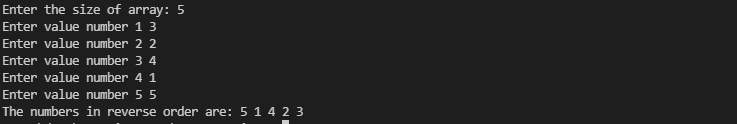Write a C++ program that asks the user to input an N number of values in an array (means, the size of the array as well as all the values should be input by the user) and then displays those values in reverse order. Sample Output

Enter the size of the array: 5

Enter value number 1 3

Enter value number 2 2

Enter value number 3 4

Enter value number 4 1

Enter value number 5 5

The numbers in reverse order are: 5 1 4 2 3

#include <iostream>
using namespace std;

int main()
{
int size;
int i = 0;

cout<<"Enter the size of the array: ";
cin>>size;
int *arr = new int[size];
for (i = 0; i < size; i++)
{
cout<<"Enter value number "<<i+1<<" ";
cin>>arr[i];
}
cout<<"The numbers in reverse order are: ";
for (i = size-1; i >= 0; i--)
{
cout<<arr[i]<<" ";
}

return 0;
}cpp programming exercise with solution